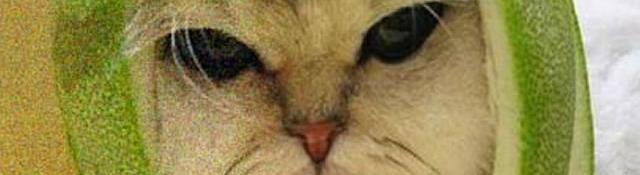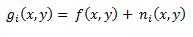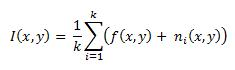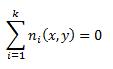## The Theory

Series: Noise reduction by averaging:

1. The Technique
2. The Theory

## Now for the theory

Pretty amazing results right? And this happened with just 25 images. Just imagine the quality of images you'd get if you just sit and take snapshots constantly (like the telescopes do).

## Probability does it all

Noise is random in nature. Its also guassian in nature. What that means is, if you had a piece of noise like:

Now if you were to sum up the intensities of each pixel and then divide it by the total number of pixels, you'll end up with a zero (or a value very much near 0).

So, if you have multiple images with different noise patterns... its very likely that averaging corresponding pixels will cause the noise to vanish.

## Mathematically...

Let gi denote each of the 25 images. So, gi is a sum of the original image (denoted by f) and some noise (denoted by ni).The operation we're performing in this technique is:We're summing up the k images (25 in our case) and dividing it by k. But we know that:So on expanding the expression for I(x,y) we end up with only f(x,y)... which is the original image!

## More images mean better noise removal

The more images you have, the better the noise removal. This sounds plausible because ideally, if you have an infinite number of cases... only then will the sum of the noise be exactly zero.

I did a little experiment on how the quality of the image improves as the number of noisy images increases. See the results for yourself:

So apparently, after a few iterations of averaging, the quality of the image reaches a plateau.

## Conclusion

So now you have a great new technique in your arsenal that you can use whenever required! If you have any doubts or criticism or suggestions, do let me know!

Have a look at the actual C implementation

## More in the series

This tutorial is part of a series called Noise reduction by averaging:

1. The Technique
2. The Theory

#### Related posts#### 7 unique neural network architectures